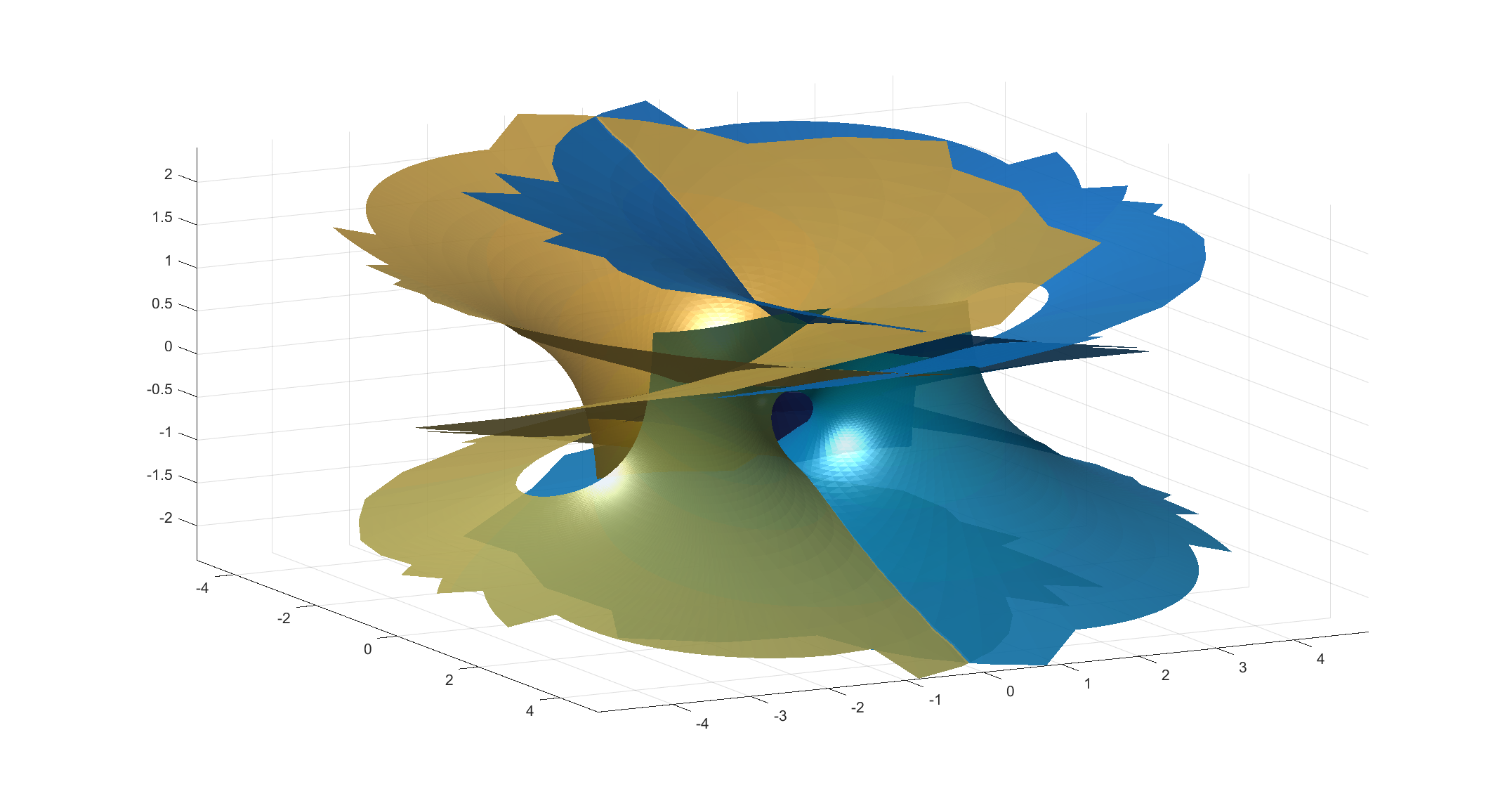# Håkan’s surface

Here is a minimal surface based on the Weierstrass-Enneper representation$f(z)=1, g(z)=\tanh^2(z)$. Written explicitly as a function from the complex number z to 3-space it is$\Re([-\tanh(z)(\mathrm{sech}^2(z)-4)/6,i(6z+\tanh(z)(\mathrm{sech}^2(z)-4))/6,z-\tanh(z)])$.Håkan’s surface, a minimal surface with Weierstrass-Enneper representation f=1,g=tanh(z)^2.

It is based on my old tanh surface, but has a wilder style. It gets helped by the fact that my triangulation in the picture is pretty jagged. On one hand it has two flat ends, but also a infinite number of catenoid openings (only two shown here).

I call it Håkan’s surface, since I came up with it on my dear husband’s birthday. Happy birthday, Håkan!